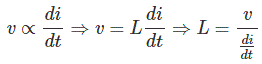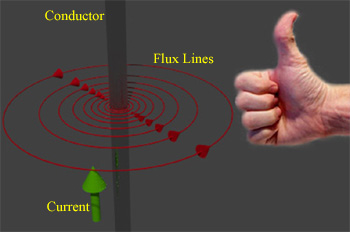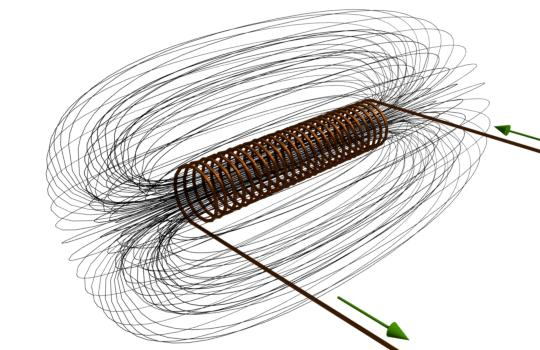# What is Inductor and Inductance | Theory of Inductor

## What is Inductor?

If a time varying current flowing through a coil there is an emf induced in it. The induced emf across the coil is directly proportional to the rate of change of current with respect to time. Due to the property inducing emf, all types of electrical coil can be referred as inductor. An inductor is an energy storage device which stores energy in form of magnetic field.

### What is Inductance?

As we already told, the induced emf across a coil is directly proportional to the rate of change of current through it. The proportionality constant in that relation is known as inductance.## Theory of Inductor

A current through a conductor produces a magnetic field surround it. The strength of this field depends upon the value of current passing through the conductor. The direction of the magnetic field is found using the right hand grip rule, which shown. The flux pattern for this magnetic field would be number of concentric circle perpendicular to the detection of current.Now if we wound the conductor in the form of a coil or solenoid, it can be assumed that there will be concentric circular flux lines for each turn of the coil as shown. But it is not possible practically, as if concentric circular flux lines for each turn exist, they will intersect each other. However, since lines of flux cannot intersect, the flux lines for an individual turn will distort to form complete flux loops around the whole coil as shown. This flux pattern of a current carrying coil is similar to a flux pattern of a bar magnet as shown.Now if the current through the coil gets changed, the magnetic flux produced by it will also get changed at the same rate. As the flux already surrounds the coil, this changing flux obviously links the coil. Now according to Faraday’s law of electromagnetic induction, if changing flux links with a coil, there would be an induced emf in it. Again as per Lenz’s law, this induced emf opposes every cause of producing it. Hence, the induced emf is in opposite of the applied voltage across the coil.

## Types of Induction

There are two types of Induction self induction and mutual induction.

### Self Induction

When time varying current flows in a coil the time varying flux is produced and this varying flux will link with that coil itself and as a result there will be emf induced in the coil itself. This type of Induction is called self induction.

### Mutual Induction

When time varying current flows in a coil it produces time varying flux as we have already told. This time varying flux may link with another nearby coil. Due to this flux linkage there will be an induced emf in the second coil. This type of electrical induction is called mutual induction. Hence mutual induction can be defined as the induction of emf in one coil due to time varying current flowing in any other nearby coil.

### Mutual Induction

The inductance of a coil due to current in another nearby coil is called mutual inductance.

### Self Inductance

The inductance of a coil or inductor due to its own current is called self inductance.

For details study of self inductance and mutual inductance, we have other articles.

Want To Learn Faster? 🎓
Get electrical articles delivered to your inbox every week.
No credit card required—it’s 100% free.